Скачать презентацию 1 -5 The distributive Property You can use

6c9f56fca1886fbfdbbbfc88d2e880f6.ppt

• Количество слайдов: 6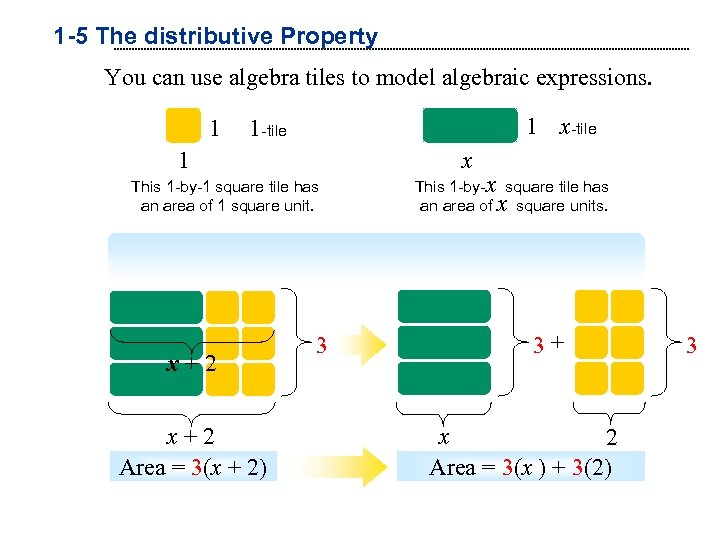1 -5 The distributive Property You can use algebra tiles to model algebraic expressions. 1 1 x-tile 1 x This 1 -by-1 square tile has an area of 1 square unit. This 1 -by-x square tile has an area of x square units. Model the Distributive Property using Algebra Tiles x+2 Area = 3(x + 2) 3 3+ x 2 Area = 3(x ) + 3(2) 3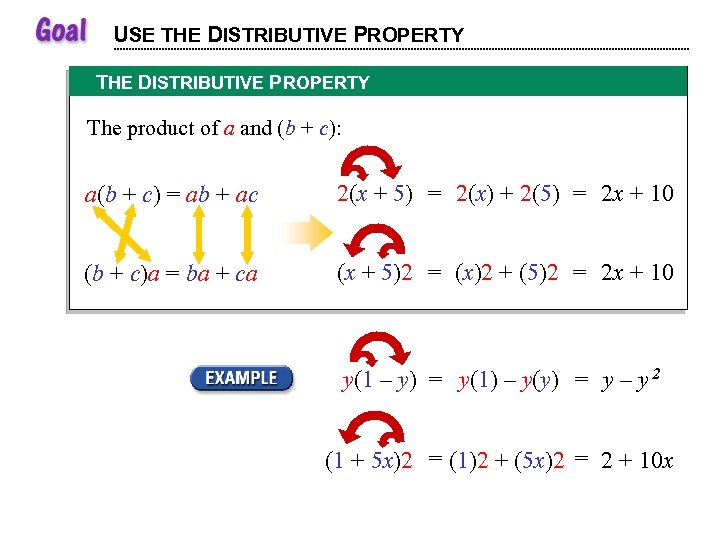USE THE DISTRIBUTIVE PROPERTY The product of a and (b + c): a(b + c) = ab + ac 2(x + 5) = 2(x) + 2(5) = 2 x + 10 (b + c)a = ba + ca (x + 5)2 = (x)2 + (5)2 = 2 x + 10 y(1 – y) = y(1) – y(y) = y – y 2 (1 + 5 x)2 = (1)2 + (5 x)2 = 2 + 10 x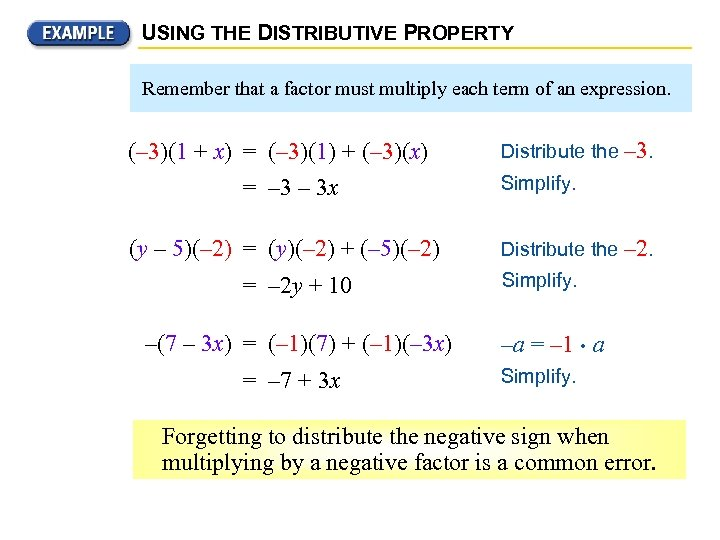USING THE DISTRIBUTIVE PROPERTY Remember that a factor must multiply each term of an expression. Distribute the – 3. (– 3)(1 + x) = (– 3)(1) + (– 3)(x) = – 3 x Simplify. (y – 5)(– 2) = (y)(– 2) + (– 5)(– 2) Distribute the – 2. = – 2 y + 10 –(7 – 3 x) = (– 1)(7) + (– 1)(– 3 x) = – 7 + 3 x Simplify. –a = – 1 • a Simplify. Forgetting to distribute the negative sign when multiplying by a negative factor is a common error.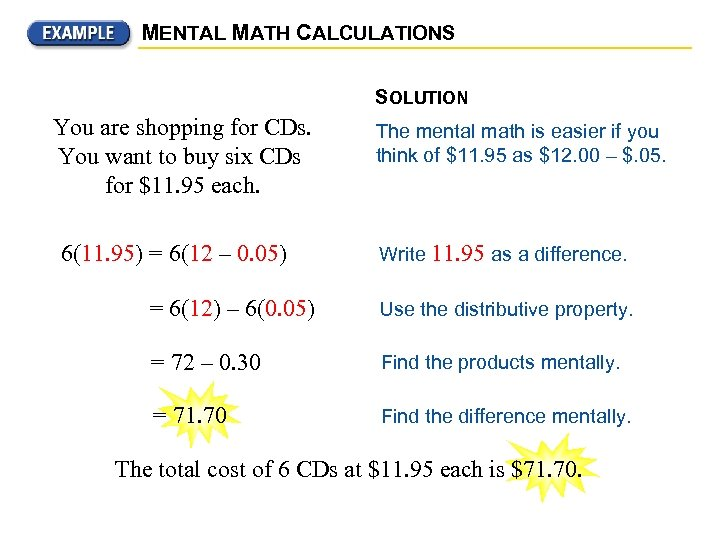MENTAL MATH CALCULATIONS SOLUTION You are shopping for CDs. You want to buy six CDs for \$11. 95 each. 6(11. 95) = 6(12 – 0. 05) The mental math is easier if you think of \$11. 95 as \$12. 00 – \$. 05. Write 11. 95 as a difference. = 6(12) – 6(0. 05) Use the distributive property to calculate the total cost = 72 – 0. 30 mentally. Use the distributive property. = 71. 70 Find the difference mentally. Find the products mentally. The total cost of 6 CDs at \$11. 95 each is \$71. 70.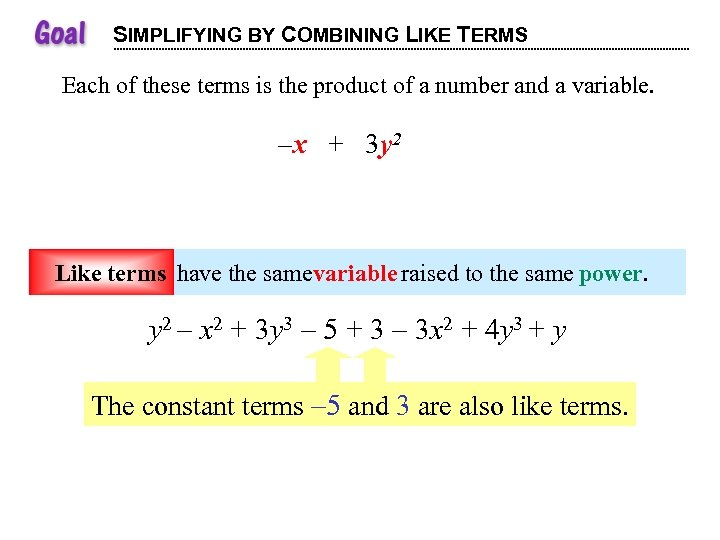SIMPLIFYING BY COMBINING LIKE TERMS Each of these terms is the product of a number and a variable. terms number variable. – x + 3 y 2 – 1 is the x is the y is the 3 coefficient ofsame variable raised to the same power. variable. x. variable. y 2. power. coefficient of Like terms have the Like terms y 2 – x 2 + 3 y 3 – 5 + 3 – 3 x 2 + 4 y 3 + y The constant terms – 5 and 3 2 also like terms. are 3 2 3 x y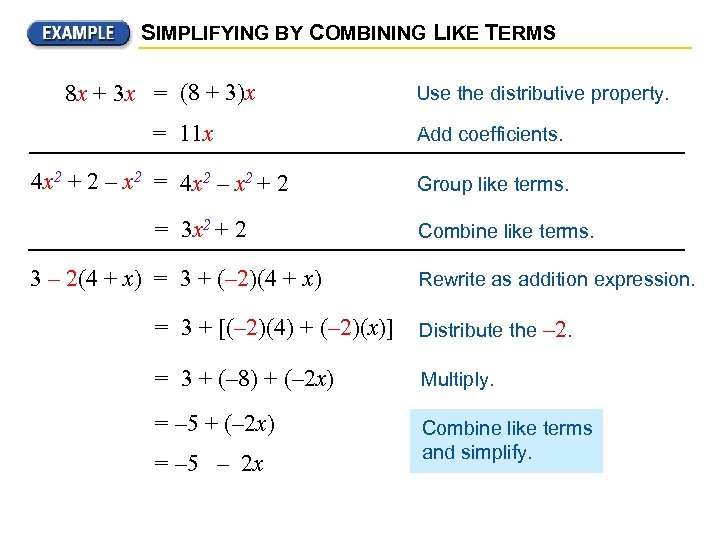SIMPLIFYING BY COMBINING LIKE TERMS 8 x + 3 x = (8 + 3)x = 11 x 4 x 2 + 2 – x 2 = 4 x 2 – x 2 + 2 = 3 x 2 + 2 3 – 2(4 + x) = 3 + (– 2)(4 + x) Use the distributive property. Add coefficients. Group like terms. Combine like terms. Rewrite as addition expression. = 3 + [(– 2)(4) + (– 2)(x)] Distribute the = 3 + (– 8) + (– 2 x) Multiply. = – 5 + (– 2 x) Combine like terms and simplify. = – 5 – 2 x – 2.